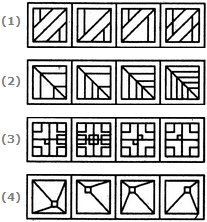# Non Verbal Reasoning - Rule Detection - Discussion

### Discussion :: Rule Detection - Section 1 (Q.No.5)

In each of the following questions, choose the set of figures which follows the given rule.

5.

Choose the set of figures which follows the given rule.

Rule: The series becomes complex as it proceeds.[A]. 1 [B]. 2 [C]. 3 [D]. 4

Explanation:

No answer description available for this question.

 Anil said: (May 7, 2011) In second case coming back from last figure at second one half portion of one tilted line being quashed which didnot follow the regular pattern.

 Ariel said: (Jun 15, 2014) The second one is the correct answer because more and more lines are added in the pattern. The first one first decrease the number of lines, so it is wrong. The third and fourth one also didn't become more complete, they even get simpler. So the correct answer is B.